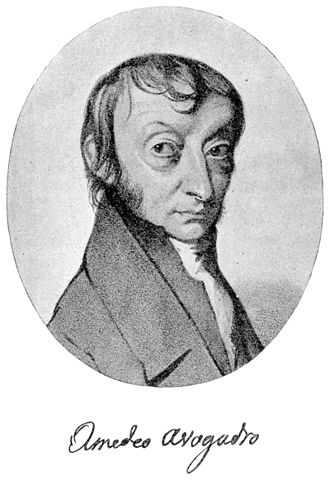Skip to main content

# 2.2.4: Avogadro's Number and the Mole

•• Contributed by Boundless
• General Microbiology at Boundless

The mole is represented by Avogadro’s number, which is 6.022 × 1023 atoms or molecules per mol.

Learning Objectives

• Define and memorize Avogadro’s number

## Key Points

• The mole allows scientists to calculate the number of elementary entities (usually atoms or molecules ) in a certain mass of a given substance.
• Avogadro’s number is an absolute number: there are 6.022 × 1023elementary entities in 1 mole. This can also be written as 6.022 × 1023mol-1.
• The mass of one mole of a substance is equal to that substance’s molecular weight. For example, the mean molecular weight of water is 18.015 atomic mass units (amu), so one mole of water weight 18.015 grams.

## Key Terms

• mole: The amount of substance of a system that contains as many elementary entities as there are atoms in 12 g of carbon-12.

The chemical changes observed in any reaction involve the rearrangement of billions of atoms. It is impractical to try to count or visualize all these atoms, but scientists need some way to refer to the entire quantity. They also need a way to compare these numbers and relate them to the weights of the substances, which they can measure and observe. The solution is the concept of the mole, which is very important in quantitative chemistry.

## Avogadro’s Number

Amadeo Avogadro first proposed that the volume of a gas at a given pressure and temperature is proportional to the number of atoms or molecules, regardless of the type of gas. Although he did not determine the exact proportion, he is credited for the idea.Figure: Amedeo Avogadro: Amedeo Avogadro is credited with the idea that the number of entities (usually atoms or molecules) in a substance is proportional to its physical mass.

Avogadro’s number is a proportion that relates molar mass on an atomic scale to physical mass on a human scale. Avogadro’s number is defined as the number of elementary particles (molecules, atoms, compounds, etc.) per mole of a substance. It is equal to 6.022 × 1023 mol-1and is expressed as the symbol NA.

Avogadro’s number is a similar concept to that of a dozen or a gross. A dozen molecules is 12 molecules. A gross of molecules is 144 molecules. Avogadro’s number is 6.022 × 1023molecules. With Avogadro’s number, scientists can discuss and compare very large numbers, which is useful because substances in everyday quantities contain very large numbers of atoms and molecules.

## The Mole

The mole (abbreviated mol) is the SI measure of quantity of a “chemical entity,” such as atoms, electrons, or protons. It is defined as the amount of a substance that contains as many particles as there are atoms in 12 grams of pure carbon-12. So, 1 mol contains 6.022×1023 elementary entities of the substance.

## Chemical Computations with Avogadro’s Number and the Mole

Avogadro’s number is fundamental to understanding both the makeup of molecules and their interactions and combinations. For example, since one atom of oxygen will combine with two atoms of hydrogen to create one molecule of water (H2O), one mole of oxygen (6.022 × 1023 of O atoms) will combine with two moles of hydrogen (2 × 6.022 × 1023 of H atoms) to make one mole of H2O.

Another property of Avogadro’s number is that the mass of one mole of a substance is equal to that substance’s molecular weight. For example, the mean molecular weight of water is 18.015 atomic mass units (amu), so one mole of water weight 18.015 grams. This property simplifies many chemical computations.

If you have 1.25 grams of a molecule with molecular weight of 134.1 g/mol, how many moles of that molecule do you have?

1.25g×1 mole134.1g=0.0093 moles.1.25g×1 mole134.1g=0.0093 moles.

The Mole, Avogadro: This video introduces counting by mass, the mole, and how it relates to atomic mass units (AMU) and Avogadro’s number.

• Was this article helpful?